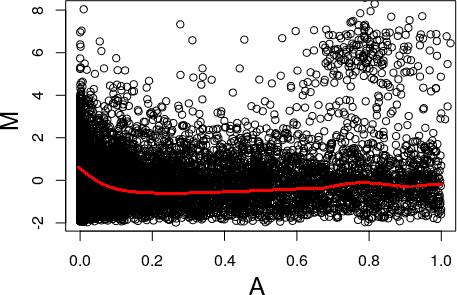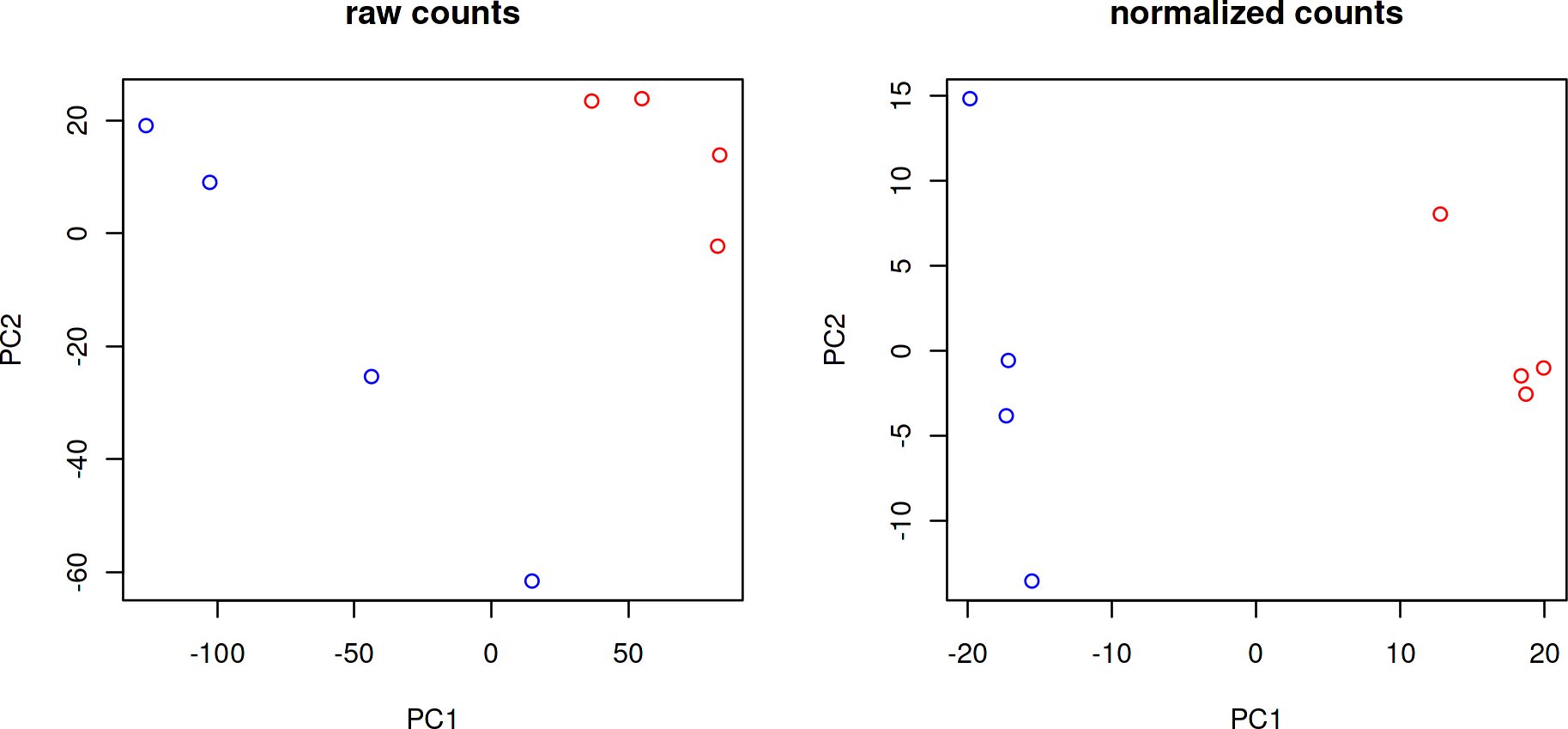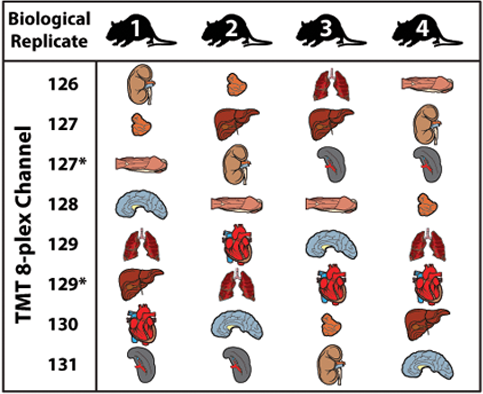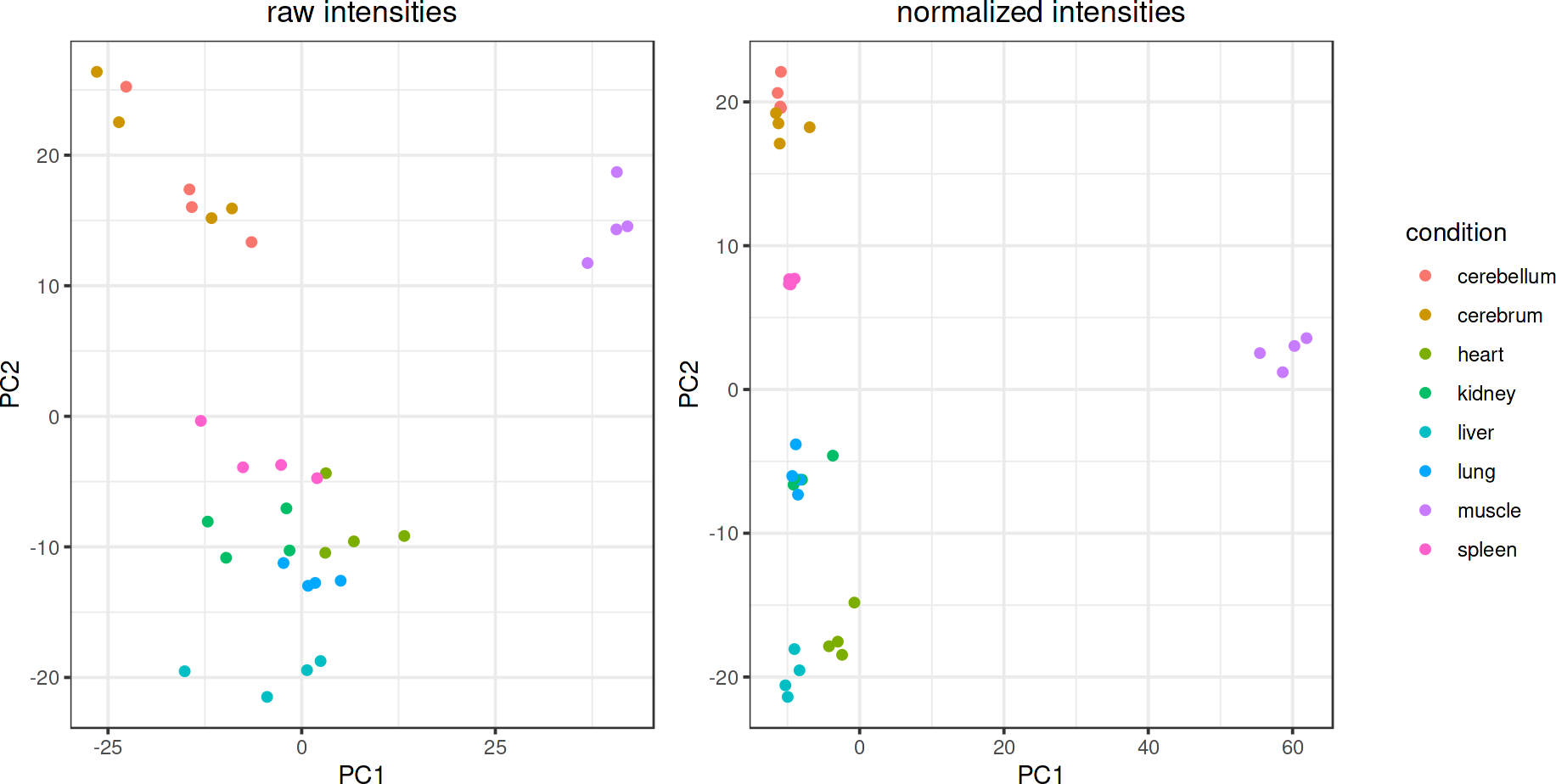# Contents

``````knitr::opts_chunk\$set(echo = TRUE,dev="CairoPNG")
library(knitr)
library(tidyr)
library(ggplot2)
library(gridExtra)
library(magick)``````
``````## Linking to ImageMagick 6.9.10.23
## Enabled features: fontconfig, freetype, fftw, lcms, pango, webp, x11
## Disabled features: cairo, ghostscript, heic, raw, rsvg``````
``## Using 2 threads``
``library(limma)``

# 1 Introduction

CONSTANd normalization has been proven to work on mass spectrometry intensity values (Maes et al.) in shotgun proteomics and on RNA-seq count data (Van Houtven et al.), but is in principle applicable to any kind of quantification matrices, for instance in other -omics disciplines. It normalizes the data matrix `data` by raking (using the RAS method by Bacharach, see references) the Nrows by Ncols matrix such that the row means and column means equal 1. The result is a normalized data matrix `K=RAS`, a product of row mulipliers `R` and column multipliers `S` with the original matrix `A`. Missing information needs to be presented as `NA` values and not as zero values, because CONSTANd is able to ignore missing values when calculating the mean.

## 1.1 Getting started

The `CONSTANd` package has to be loaded to use the function. To load `CONSTANd`, type the following command after launching R.

``````#install.packages("BiocManager")
#BiocManager::install("CONSTANd")
library(CONSTANd)``````

## 1.2 Data assumptions

To warrant that the normalization procedure itself is not biased, three assumptions about the data are made (as for any type of data-driven normalization method) which may be verified by making MA-plots (see examples in section 5):

• The majority of features (peptides/genes/…) are not differentially expressed, to avoid a bias in the estimate of the mean value. It is assumed that up-down shifts are not due to biological causes. The reference set used in the normalization step is the set of all peptides identified in the experiment.
MA-plot: the observations form a single ‘cloud’ with a dense center and less dense edges, as opposed to, for instance, two clouds or a cloud with uniform density.
• The number of up-regulated features is roughly equal to the number of down-regulated ones. If the data were skewed, this would lead to a bias in the normalization result.
MA-plot: the cloud of observations exhibits a bilateral symmetry about some axis (usually horizontal, but inclinations may occur).
• Any systematic bias present is linearly proportional to the magnitude of the quantification values. Only this way it is possible to find one appropriate normalization factor for each quantification sample.
MA-plot: the axis of bilateral symmetry is a straight line (which may be inclined), i.e., the moving average M-values of the central cloud form an approximately straight line.

### 1.2.1 Example of a bad MA plot

The following MA plot violates all three assumptions. In case your MA plots exhibit any of such characteristics, it is not advised to use CONSTANd - or any other data-driven method - to normalize your data, and to instead use advanced model-based approaches or, if appropriate, clean your data first.Figure 1: This MA plot violates all assumptions: there is more than one cloud, there are more upregulated entities, and the bias changes strongly in the region with small magnitudes (A-values)

# 2 Normalizing a data matrix (single assay)

The data matrix is an `Nrows` by `Ncols` matrix (e.g. an `assay` from a `SummarizedExperiment`), representing quantification values of any kind, as long as they form an identically processed (sub)set (IPS). An IPS consists of measurements from biological samples who have been processed from biological harvesting of the tissue to the detection and quantitation of the features of interest (RNA, proteins, …) in near-identical fashion. In practice, this means that there exist no factors (except those of biological interest) which may cause a systematic bias in the quantification values. One _counter_example in proteomics is samples measured on a different day or a different instrument, one _counter_example in RNA-seq would be samples measured after using different library preparation protocols. In the next section, we demonstrate how in such such cases the IPSs are normalized separately as if they were individual assays (see this section), after which they may be re-combined.

First, let’s load the ‘Leishmania’ mRNA-level data set from GEO (Gene Expression Omnibus) with identifier GSE95353. You can either download and unzip it yourself, are use the file that comes with this package.

``leish <- read.csv("../inst/extdata/GSE95353_SL_SEQ_LOGSTAT_counttable.txt" , sep='\t')``

We only need the LOG and STAT samples, which correspond to biological quadruplicates of Leishmania in two lifestages (LOG and STAT).

``````rownames(leish) <- leish\$gene
leish <- leish[, c("SL.LOG1", "SL.LOG2", "SL.LOG3", "SL.LOG4",
"SL.STAT1", "SL.STAT2", "SL.STAT3", "SL.STAT4")]
conditions <- c(rep("LOG", 4), rep("STAT", 4))``````

Let’s remove genes with low count numbers (`median > 0`) for good practice, and make sure that zero counts are replaced by `NA` to use CONSTANd.

``````# first set NA to 0 for calculating the row medians
leish[is.na(leish)] <- 0
leish <- leish[apply(leish, 1, median, na.rm = FALSE) > 0,]
leish.norm <- CONSTANd(leish)\$normalized_data``````
``````## Warning in CONSTANd(leish): Zeros in quantification matrix detected; replacing
## with NA.``````

Let’s make a PCA plot (optionally impute `NA` as zero) before and after normalization to see if it was successful:

``````leish[is.na(leish)] <- 0
# scale raw data
leish.pc.raw <- prcomp(x=t(leish), scale. = TRUE)
leish.norm[is.na(leish.norm)] <- 0
# CONSTANd already scaled the data
leish.pc.norm <- prcomp(x=t(leish.norm))
par(mfrow=c(1,2))
colors = ifelse(conditions == 'LOG', 'blue', 'red')
plot(leish.pc.raw\$x[,'PC1'], leish.pc.raw\$x[,'PC2'],
xlab='PC1', ylab='PC2', main='raw counts', col=colors)
plot(leish.pc.norm\$x[,'PC1'], leish.pc.norm\$x[,'PC2'],
xlab='PC1', ylab='PC2', main='normalized counts', col=colors)``````Figure 2: LOG samples (blue) are separated more clearly by PC1 from the STAT samples (red) in the Leishmania data set after CONSTANd normalization

Indeed, the PCA plot indicates that the first principal component, which is the direction along which there is most varability in the measurements, successfully defines a separation between the biological conditions. In other words: after normalization the biological differences between the samples dominate any other sources of systematic bias.

# 3 Normalization across multiple assays

Here, we demonstrate multiple IPSs involved in one experiment may be normalized separately as if they were individual assays (see previous section), after which they may be re-combined for joint analysis. We demonstrate CONSTANd normalization across multiple assays using the Organs example data set from QCQuan, a proteomics webtool built around the CONSTANd algorithm. The Organs data set entails samples from 8 different mouse organs in biological quadruplicate, whose peptides have been measured in 4 different instrument runs (1 per mouse) using TMT-labeled (8-plex) tandem mass spectrometry.Figure 3: Organs data set experimental setup: 8 mouse organ samples spread across 4 TMT 8-plex tndem-MS runs, one for each mouse
Image source: Bailey, Derek J., et al. “Intelligent data acquisition blends targeted and discovery methods.” Journal of proteome research 13.4 (2014): 2152-2161. https://doi.org/10.1021/pr401278j.

You can either download the original data from the web, unzip it and set `LOAD_ORIGINAL=TRUE`, or load the cleaned data from this package.

``````LOAD_ORIGINAL = FALSE
# load extra functions to clean the original data and study design files
source('../inst/script/functions.R')
organs <- list(BR1, BR2, BR3, BR4)
organs <- clean_organs(organs)
} else {  # load cleaned file that comes with this package
}
# construct the study design from the QCQuan DoE file and arrange it properly
study.design <- rearrange_organs_design(study.design)``````

The data is now cleaned up just enough to demonstrate the effect of normalization. In a proper analysis one may want to do additional checks, cleaning, and summarization steps. However, none of those affect the use of CONSTANd and one can use CONSTANd on the PSM, peptide or protein level, although the outcome may of course differ.

``````# the data has been summarized to peptide level
``````##  "AAAPAPEEEMDECEQALAAEPK"         "AAASTDYYK"
##  "AAESSAMAATEK"                   "AAFDDAIAELDTLSEESYKDSTLIMQLLR"``````
``````# only the quantification columns were kept, which here happen to be all of them
quanCols <- colnames(organs[])
quanCols``````
``##  "126"  "127N" "127C" "128C" "129N" "129C" "130C" "131"``
``````organs <- lapply(organs, function(x) x[,quanCols])
# make unique quantification column names
for (i in seq_along(organs)) {
colnames(organs[[i]]) <- paste0('BR', i, '_', colnames(organs[[i]])) }``````

In MS-based proteomics, anlyzing samples even with the same intrument on the same day but in a different run can cause systematic bias between the runs. Therefore, we normalize each BR run dataframe independently:

``````organs.norm.obj <- lapply(organs, function(x) CONSTANd(x))
organs.norm <- lapply(organs.norm.obj, function(x) x\$normalized_data)``````

However, after CONSTANd normalization these values become comparable. We demonstrate this again using PCA plots, after merging the non-normalized data frames and merging the normalized data frames. Let’s prepare the merge by doing some administration on our column names.

``````merge_list_of_dataframes <- function(list.dfs) {
return(Reduce(function(x, y) {
tmp <- merge(x, y, by = 0, all = FALSE)
rownames(tmp) <- tmp\$Row.names
tmp\$Row.names <- NULL
return(tmp)
}, list.dfs))}
organs.df <- merge_list_of_dataframes(organs)
organs.norm.df <- merge_list_of_dataframes(organs.norm)``````

We can now make PCA plots using data from all 4 runs:

``````# PCA can't deal with NA values: impute as zero (this makes sense in a multiplex)
organs.df[is.na(organs.df)] <- 0
organs.norm.df[is.na(organs.norm.df)] <- 0
# scale raw data
organs.pc <- prcomp(x=t(organs.df), scale. = TRUE)
# CONSTANd already scaled the data
organs.norm.pc <- prcomp(x=t(organs.norm.df))``````

The randomized study design and separate design file makes coloring the PCA plot a bit tedious.

``````# prepare plot color mappings
organs.pcdf <- as.data.frame(organs.pc\$x)
organs.pcdf <- merge(organs.pcdf, study.design, by=0)
rownames(organs.pcdf) <- organs.pcdf\$Row.names
organs.norm.pcdf <- as.data.frame(organs.norm.pc\$x)
organs.norm.pcdf <- merge(organs.norm.pcdf, study.design, by=0)
rownames(organs.norm.pcdf) <- organs.norm.pcdf\$Row.names``````Figure 4: Before normalization, the samples in the Organs data set are all scattered across the PCA plot
After normalization, they are correctly grouped according to biological condition, even though all 4 samples in each group have been measured in a different MS run. The big separation in PC1 suggests that the muscle tissue is very different from the tissues of other types of organs!

# 4 Optional arguments

## 4.1 target

The variable `target=1` sets the mean quantification value in each row and column during the raking process. After normalization, each row and column mean in the matrix will be equal to `target` up to a certain `precision` (see further). Setting a custom value does not affect the normalization procedure, but does scale the output values. Caution: quantification values from matrices normalized with different target values may under normal circumstances not be directly compared. Change this value only when you need to and when you know what you are doing.

## 4.2 maxIterations and precision

The variable `maxIterations=50` is an integer value that defines a hard stop on the number of raking cycles, forcing the algorithm to return even if the desired precision was not reached (a warning will be printed). The variable `precision=1e-5)` defines the stopping criteria based on the L1-norm as defined by Friedrich Pukelsheim, Bruno Simeone in “On the Iterative Proportional Fitting Procedure: Structure of Accumulation Points and L1-Error Analysis”.

# 5 Making MA plots

We recommend building upon the following function to make MA plots when using CONSTANd.

``````MAplot <- function(x,y,use.order=FALSE, R=NULL, cex=1.6, showavg=TRUE) {
# make an MA plot of y vs. x that shows the rolling average,
M <- log2(y/x)
xlab = 'A'
if (!is.null(R)) {r <- R; xlab = "A (re-scaled)"} else r <- 1
A <- (log2(y/r)+log2(x/r))/2
if (use.order) {
orig.order <- order(A)
A <- orig.order
M <- M[orig.order]
xlab = "original rank of feature magnitude within IPS"
}
# select only finite values
use <- is.finite(M)
A <- A[use]
M <- M[use]
# plot
print(var(M))
plot(A, M, xlab=xlab, cex.lab=cex, cex.axis=cex)
# rolling average
if (showavg) { lines(lowess(M~A), col='red', lwd=5) }
}``````

When verifying that input data adheres to the assumptions in section 1.2, one could use this function (preferably on each pair of biological conditions in each IPS) without optional arguments:

``````# Example for cerebrum and kidney conditions in run (IPS) 1 in the Organs data set.
cerebrum1.colnames <- rownames(study.design %>% dplyr::filter(run=='BR1', condition=='cerebrum'))
kidney1.colnames <- rownames(study.design %>% dplyr::filter(run=='BR1', condition=='kidney'))
cerebrum1.raw <- organs.df[,cerebrum1.colnames]
kidney1.raw <- organs.df[,kidney1.colnames]
# rowMeans in case of multiple columns
if (!is.null(dim(cerebrum1.raw))) cerebrum1.raw <- rowMeans(cerebrum1.raw)
if (!is.null(dim(kidney1.raw))) kidney1.raw <- rowMeans(kidney1.raw)
MAplot(cerebrum1.raw, kidney1.raw)``````
``##  1.557956``